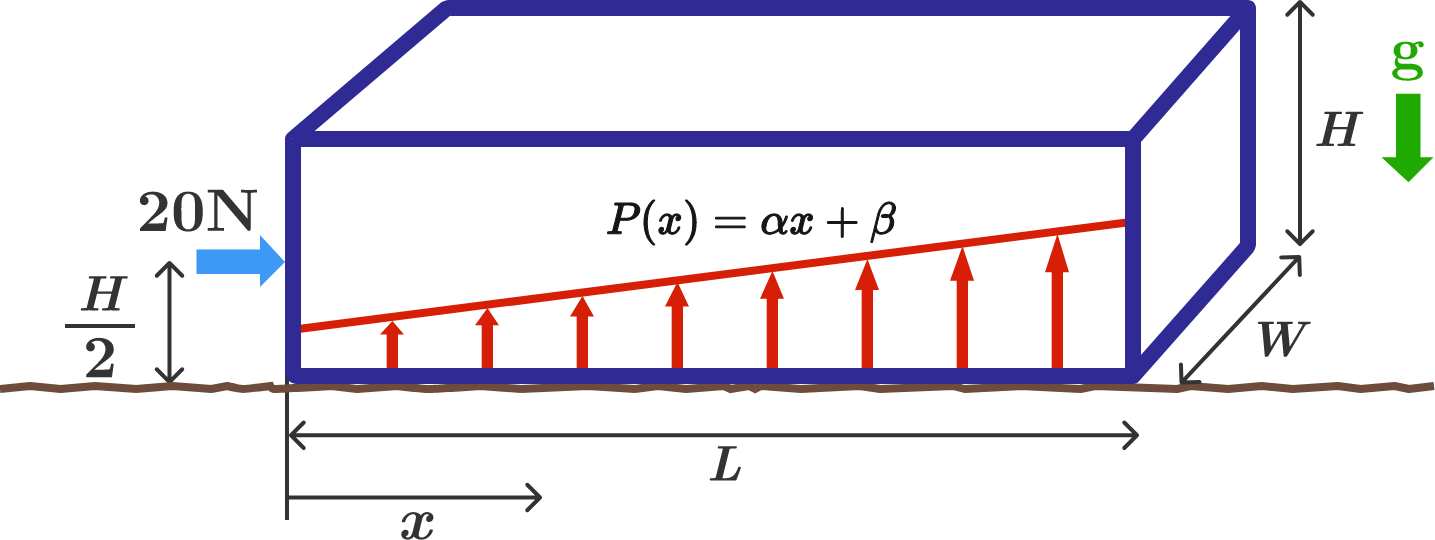# Pressure ProfileThere is a $10 \text{ kg}$ box of length, height, and width $(L = 2\text{ m}, H = 0.5\text{ m}, W = 1\text{ m})$ sitting on a rough surface.

A $20\text{ N}$ rightward horizontal force is applied at the middle of the left side of the box at a distance of $\frac{H}{2}$ from the bottom of the box. There is sufficient friction that the box remains stationary.

Let the variable $x$ represent the horizontal position (in meters) relative to the left side of the box. With the horizontal force applied, the upward pressure $\big($in $\text{N/m}^{2}\big)$ associated with the normal reaction varies according to the equation $P(x) = \alpha x + \beta$.

Determine $\frac{\beta}{\alpha}$, to 2 decimal places.

Details and Assumptions:

• There is a uniform downward gravitational acceleration of $10\text{ m/s}^{2}$.
• Assume that the box is solid and has uniform mass density.
• Linear pressure variation is an assumption made by the problem author. See here for further discussion.
×